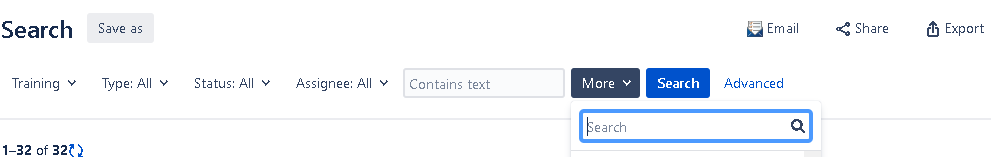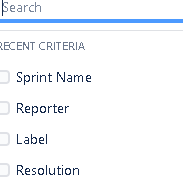cancel
Showing results for
Did you mean:
See all
See all
##### Top groups
Explore all groups

## Come for the products,stay for the community

The Atlassian Community can help you and your team get more value out of Atlassian products and practices.4,359,759
Community Members

Community Events
168
Community Groups

Hello,

I Try to build a new calculated measure to sum time spent on issues during a sprint, I took inspiration from sprint burn down exemple:

https://docs.eazybi.com/display/EAZYBIJIRA/Sprint+burn-down+or+burn-up+chart

Then I adapted the "Cumulative Story Points resolved" calculated measure, but I have an issue because the cumulative on the first day of the sprint is not equal to the hours spent on that dayHere is my code :

```CASE
WHEN NOT [Time].CurrentHierarchyMember IS [Time].DefaultMember
THEN
Sum(
{ [Time].CurrentHierarchyMember.Parent.Level.Members.Item(0):
[Time].CurrentHierarchyMember.Parent.PrevMember },
[Measures].[Hours spent]
) +
Sum(
PeriodsToDate([Time].CurrentHierarchyMember.Parent.Level),
[Measures].[Hours spent]
)
WHEN NOT [Sprint].CurrentMember IS [Sprint].DefaultMember
THEN
Sum(
{ [Sprint].CurrentMember.Level.Members.Item(0):
[Sprint].CurrentMember },
[Measures].[Hours spent]
)
END```

If anybody can help me on this point, I will be greatfull.

Best regards,

#### 1 accepted

This formula will calculate cummulative hours spent on issues in selected sprint since beginning of time. It seems that in your case hours were spent on issues in this sprint already before beginning of this sprint therefore on first day it already shows much higher amount of cummulative hours spent.

If you want to calculate cummulative hours spent just within sprint dates then you can define "Cumulative Time Spent" with formula:

```Sum(
Filter(
[Time].CurrentHierarchyMember.Level.Members,
[Measures].[Time within Sprint] = 1
).Item(0):[Time].CurrentHierarchyMember,
[Measures].[Hours spent]
)```

It will filter only Time periods within current sprint (using the same "Time within Sprint" measure) and then will calculate cumulative sum from first sprint Time period until current.

Thks for the tips Raimonds, it is exactly what I needed.

Best regards,

Is there any way I can add any more customized search criteria in my search bar ?